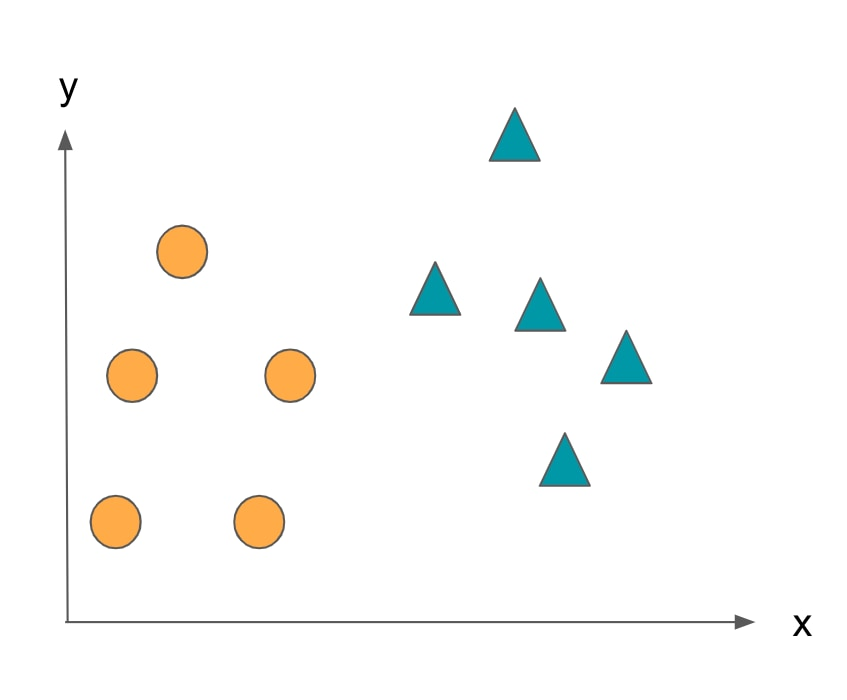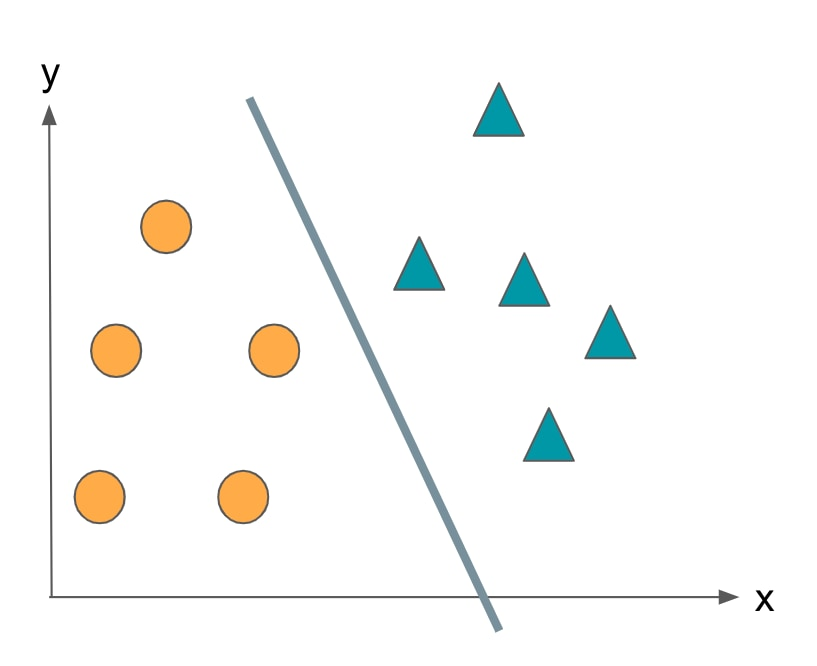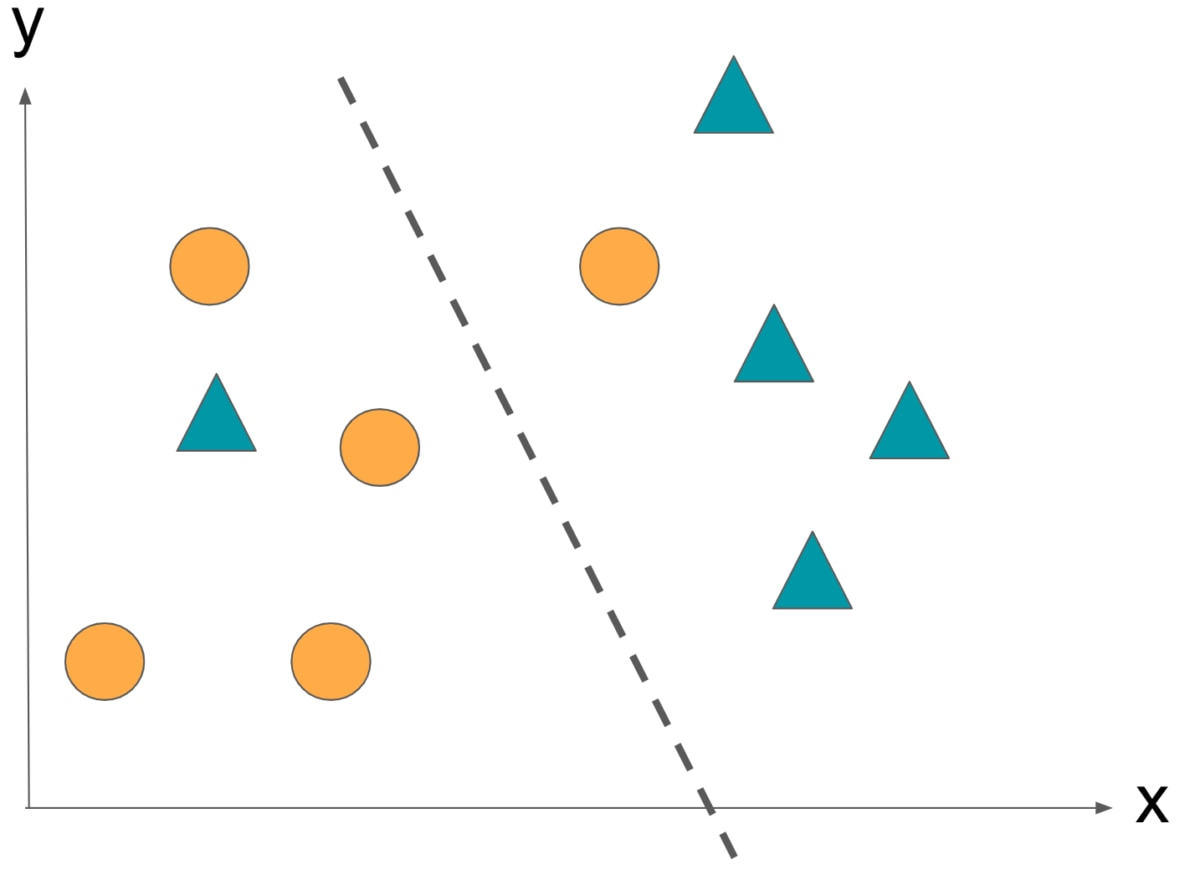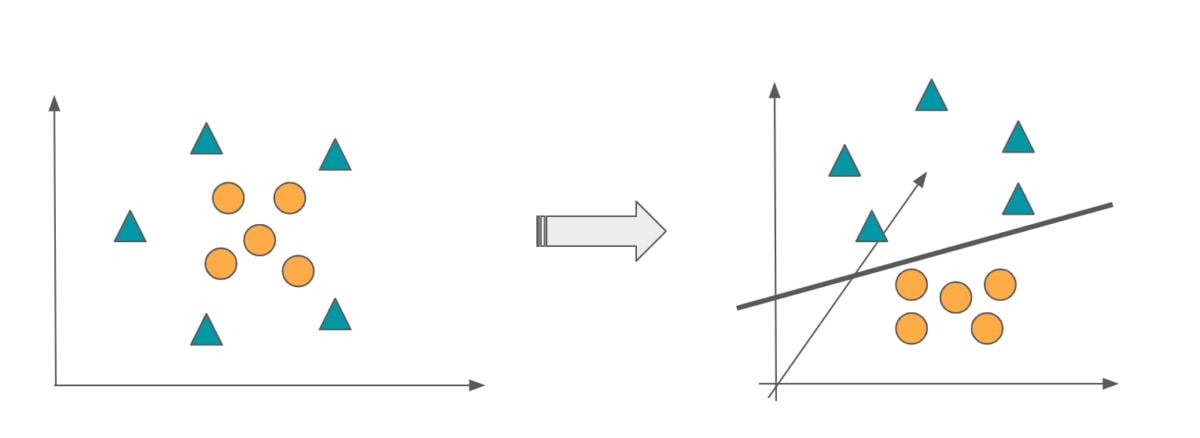Saturday, December 2, 2023
HomeAIWhat is SVM (Support Vector Machine)?

# What is SVM (Support Vector Machine)?

Contents [ Hide ]

• What is SVM (Support Vector Machine)?
• How SVMs work
• merit
• Demerit
• What you can do with SVM [3 application examples]
• 1. Stock price forecast
• 2. Digit recognition
• 3. Outlier detection
• Let’s implement SVM!
• Learning SVM -recommendations and notes-
• Recommended study method
• Notes on learning
• summary

## What is SVM (Support Vector Machine)?

First, let me explain what support vectors are.

A support vector is “the data closest to the straight line that divides the data”. It uses a concept called “margin maximization” to find the correct classification criteria.

Consider the problem of classifying with two features. Margin is the distance between the border and the data. If this is too small, a small difference in data will result in an erroneous decision.

The goal of SVM is to draw a border that maximizes the margin. Clearly, data near boundaries are data that are difficult to classify.

On the other hand, we don’t need to consider data that are far from the boundary (obvious classification). Therefore, we only use the data near the boundary, i.e. the support vectors, for classification.

### How SVMs work

Based on the two features x and y, create a classifier that classifies the data into either the ◯ or △ class.Find the boundary line that best divides this training data, taking into account the class of each data.All data located on one side of this boundary are classified as ◯, and all data located on the other side are classified as △.Now, which is the better boundary, red or blue?

The answer is red. You can see that the blue border has a smaller margin. The above is how to determine the boundary of the SVM.

#### overfitting problem

So far, we have discussed examples where a boundary line clearly divides a data group into two. In this way, a margin based on the assumption that the two can be clearly separated is called a hard margin.

On the other hand, for data that is difficult to clearly divide into two types and inevitably results in misclassification, the soft margin is a margin that allows misclassification.

If you seek too much “accurate discrimination”, you may lose sight of valuable discriminant features, resulting in poor prediction accuracy. This problem is called overfitting.The ability to make predictions without overfitting is called generalizability. In order to increase this generalization, we dare to allow misclassification.

The soft margin satisfies “Boundaries and data are as small as possible and misclassification is as small as possible”.

The methods described so far can only handle data that can be classified by straight lines. Here, by using a technique called the ” kernel method “, it becomes possible to classify data that cannot be classified by straight lines.

We map the real-space data to a space separable by hyperplanes and then separate the data using the same mechanism as linear SVM. In the example below, objects that cannot be classified in two dimensions are mapped to three dimensions and classified.### Demerit

It is necessary to decide which algorithm to use by considering the above typical merits and demerits.

## What you can do with SVM [3 application examples]

Next, I will introduce three main application examples of SVM.

### 1. Stock price forecast

SVM (Support Vector Machine) is good at linear classification of two classes. With SVM, you can predict whether the stock price will rise or fall.

By learning past stock price fluctuation data and recognizing patterns when stock prices rise and fall compared to the previous day, you can build a machine learning model that predicts stock price movements for the next day.

### 2. Digit recognition

You can also classify images of handwritten numbers into number categories such as 0-9.

Specifically, the numbers are 10 characters from 0 to 9, which are used to identify the SVM by extending it so that it can identify multiple classes. By applying this, it can be used for things such as zip code recognition.

### 3. Outlier detection

In this case, we apply SVM to unsupervised one-class classification.

By learning one class as normal data and determining the discrimination boundary, outliers are detected based on that boundary.

It can detect fraudulent credit card transactions or detect heat and size anomalies in factories.

## Let’s implement SVM!

Here, we will implement it using scikit-learn.

scikit-learn is a Python machine learning module that is often used in SVM implementations. Here we actually refer to the official scikit-learn code.

You can train a discriminator with this code. X is the explanatory variable and y is the objective variable.

In this code, there are two 2D data, (0,0) and (1,1). The label at (0,0) is 0, indicating that the label at coordinates (1,1) is 1.

Then fit() instructs learning.

For example, if you want to classify flowers, put the characteristics such as color, size, and shape in X and the type of flower in y.

This code makes an estimation on the test data. Guess which label (2,2) will be.

In other words, if the array () and 1 label are attached here, it is successful.

## Learning SVM -recommendations and notes-

Here are some recommended study methods and points to note about SVM.

### Recommended study method

The recommended way to study is to use a school or an online course.

This time, only the basic part of how SVM works has been explained. However, it will be a high hurdle to understand in more detail.

Therefore, I think that learning at a school or online course, where questions and support systems are in place, is the fastest and correct way to learn.

For those who just want a basic understanding as one of the algorithms, an information sharing service such as Qiita is sufficient. However, we recommend that you keep in mind the following points and proceed with your study.

### Notes on learning

SVM is a very powerful algorithm that excels at classification problems. However, no matter how good the algorithm is, it is not perfect.

To use it successfully, you need to understand the problem you want to solve and decide if SVM is the best algorithm for you.

So make sure you know the pros and cons. If you understand the merits and demerits firmly, it will be useful for algorithm selection, so you will be able to learn more constructively.

## Summary

This time, I explained the basics of SVM, how to implement it, and how to study it.

I would appreciate it if you could deepen your understanding of SVM (Support Vector Machine) even a little. Thank you for watching until the end.

RELATED ARTICLES